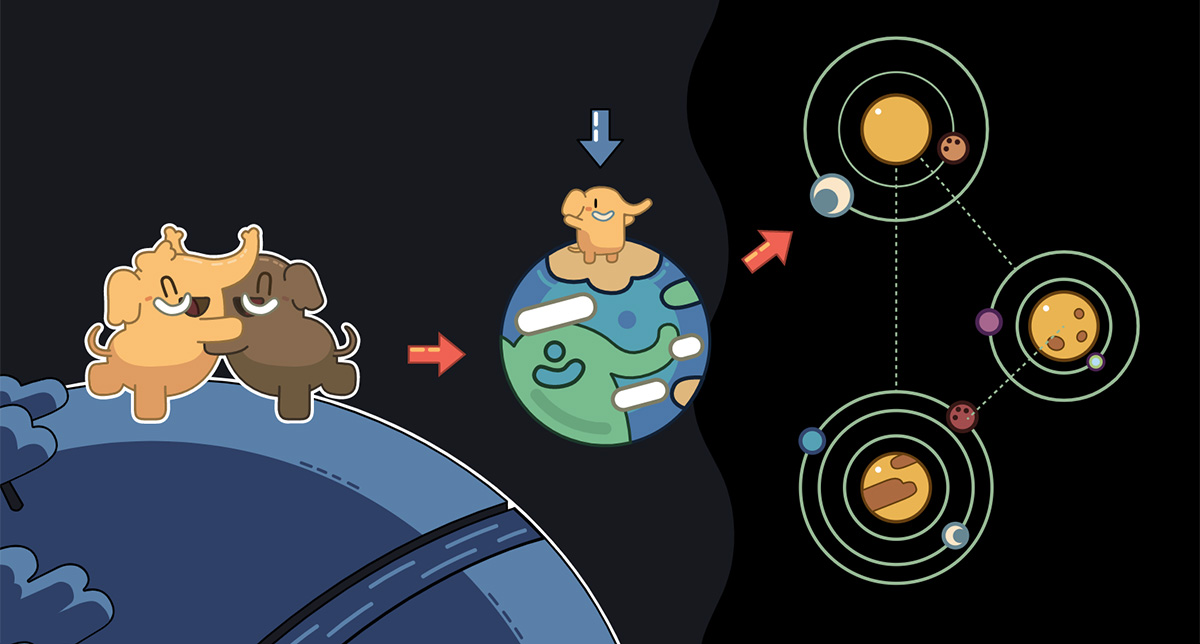Noise Bullseye

Experimenting with semi-random noise in Processing. In this generative art piece I programmed it so that each pixel has a random lightness and the lightness is biased towards a certain value. That value is determined by the sine function, which is plotted in polar coordinates.

You'll notice that there are rings of pure black... This is due to the value of the sine function being zero (I used sin( r/20 ) + 1 so the the area where the sine is zero is stretched out) and the fact that the lightness of each pixel is obtained by multiplying by the sine function. So the lightness of these pixels is a random value multiplied by the sine at that location, which ends up being zero.

I like how the result ended up looking like a 3D styrofoam bullseye.